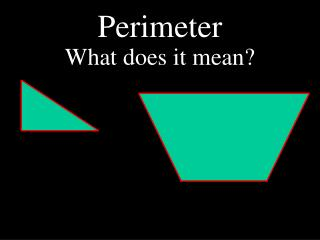DownloadDownload PresentationPerimeter

# Perimeter

Download Presentation## Perimeter

- - - - - - - - - - - - - - - - - - - - - - - - - - - E N D - - - - - - - - - - - - - - - - - - - - - - - - - - -
##### Presentation Transcript

1. Perimeter What does it mean?

2. Let’s find the perimeter. 12 ft 8 ft 10 ft

3. Let’s find the perimeter. 15 in 20 in 20 in 10 in

4. Let’s find the perimeter. This is a regular polygon. What is a regular polygon? A regular polygon is one in which all sides are the same length. So, if one of this polygon’s sides measures 5 cm... …what would the perimeter of this polygon be?

5. Let’s find the perimeter. 5 cm 5 cm 5 cm 5 cm 50 cm 5 cm 5 cm 5 cm 5 cm 5 cm 5 cm

6. So, how would you find the perimeter of this rectangle? length ??? width length

7. So, how would you find the perimeter of this rectangle? length width width length

8. So, how would you find the perimeter of this rectangle? length length + length + width + width = 2 x length + 2 x width = width width 2l + 2w = length

9. So, how would you find the perimeter of this rectangle? 7 mm ??? 5 mm 7 mm

10. So, how would you find the perimeter of this rectangle? 7 mm 2l + 2w = 2 x 7 + 2 x 5 = 5 mm 5 mm 14 + 10 = 24 cm 7 mm

11. Find the Perimeter 10 ft 2l + 2w = 2 x 10 + 2 x 8 = 0 8 ft 8 ft 20 + 16 = 36 ft 10 ft

12. Find the Perimeter 3 in 2l + 2w = 2 x 7 + 2 x 3 = 14 + 6 = 20 in 7 in ??? ???

13. Rectangle Perimeter Formula 2 in 2l + 2w = perimeter of a rectangle 2 x length + 2 x width 2l + 2w = 6 in 2 x 6 + 2 x 2 = 12 + 2 x 2 = 16 in 12 + 4 =

14. Find the Perimeter Use: 2l + 2w = perimeter of a rectangle 10 yds 1 yds 2l + 2w = Step 1: Write the formula. 2 x 10 + 2 x 1 = Step 2: Replace the variables. 20 + 2 = Step 3: Multiply first. 20 + 2 = 22 Step 4: Add last. 22 yds Step 5: Write the answer with the correct units.

15. Find the Perimeter Use: 2l + 2w = perimeter of a rectangle 9 in 3 in 2l + 2w = Step 1: Write the formula. 2 x 9 + 2 x 3 = Step 2: Replace the variables. 18 + 6 = Step 3: Multiply first. 18 + 6 = 24 Step 4: Add last. 24 in Step 5: Write the answer with the correct units.

16. Try one with someone at your table. Use: 2l + 2w = perimeter of a rectangle 4 cm 8 cm 2l + 2w = Step 1: Write the formula. 2 x 8 + 2 x 4 = Step 2: Replace the variables. 16 + 8 = Step 3: Multiply first. 16 + 8 = 24 Step 4: Add last. 24 cm Step 5: Write the answer with the correct units.

17. So, how would you find the perimeter of this square? side side side side

18. So, how would you find the perimeter of this square? side side + side + side + side = 4 x side = side 4s = side side

19. So, how would you find the perimeter of this square? 7 mm 4s = 4 x 7 = 28 mm 7 mm 7 mm 7 mm

20. So, how would you find the perimeter of this square? 4s = 5 ft 4 x 5 = 20 ft 5 ft 5 ft 5 ft

21. Square Perimeter Formula 4s = perimeter of a square 3 in 4 x side 4s = 12 in 4 x 3 =

22. Find the Perimeter Use: 4s = perimeter of a square 10 yds 4s = Step 1: Write the formula. 4 x 10 = Step 2: Replace the variables. 40 yds 4 x 10 = Step 3: Multiply and write the correct units in your answer.

23. Find the Perimeter Use: 4s = perimeter of a square 9 cm 4s = Step 1: Write the formula. 4 x 9 = Step 2: Replace the variables. 36 cm 4 x 9 = Step 3: Multiply and write the correct units in your answer.

24. Try one with someone at your table. Use: 4s = perimeter of a square 6 mm 4s = Step 1: Write the formula. 4 x 6 = Step 2: Replace the variables. 24 mm 4 x 6 = Step 3: Multiply and write the correct units in your answer.

25. Let’s Review • Perimeter is the distance around the edge of an object. What is the formula for finding the perimeter of a rectangle? What does perimeter mean? 2l + 2w What is the formula for finding the perimeter of a square? 4s

26. Now it is your turn.